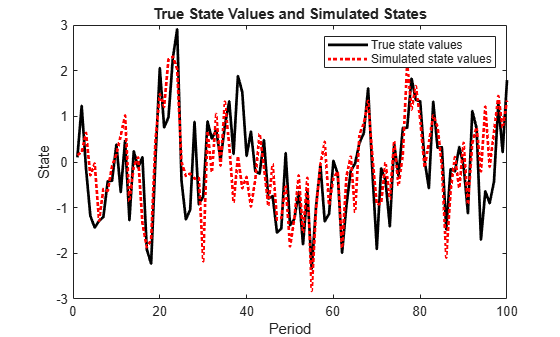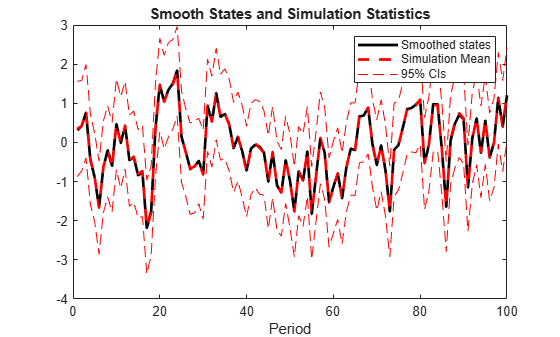# simsmooth

Class: ssm

State-space model simulation smoother

## Syntax

``X = simsmooth(Mdl,Y)``
``X = simsmooth(Mdl,Y,Name,Value)``

## Description

example

````X = simsmooth(Mdl,Y)` returns simulated states (`X`) by applying a simulation smoother to the time-invariant or time-varying state-space model (`Mdl`) and responses (`Y`). That is, the software uses forward filtering and back sampling to obtain one random path from the posterior distribution of the states.```

example

````X = simsmooth(Mdl,Y,Name,Value)` returns simulated states with additional options specified by one or more `Name,Value` pair arguments.```

## Input Arguments

expand all

Standard state-space model, specified as an`ssm` model object returned by `ssm` or `estimate`. A standard state-space model has finite initial state covariance matrix elements. That is, `Mdl` cannot be a `dssm` model object.

If `Mdl` is not fully specified (that is, `Mdl` contains unknown parameters), then specify values for the unknown parameters using the `'``Params``'` `Name,Value` pair argument. Otherwise, the software throws an error.

Observed response data, specified as a numeric matrix or a cell vector of numeric vectors.

• If `Mdl` is time invariant with respect to the observation equation, then `Y` is a T-by-n matrix, where each row corresponds to a period and each column corresponds to a particular observation in the model. T is the sample size and m is the number of observations per period. The last row of `Y` contains the latest observations.

• If `Mdl` is time varying with respect to the observation equation, then `Y` is a T-by-1 cell vector. Each element of the cell vector corresponds to a period and contains an nt-dimensional vector of observations for that period. The corresponding dimensions of the coefficient matrices in `Mdl.C{t}` and `Mdl.D{t}` must be consistent with the matrix in `Y{t}` for all periods. The last cell of `Y` contains the latest observations.

`NaN` elements indicate missing observations. For details on how the Kalman filter accommodates missing observations, see Algorithms.

### Name-Value Arguments

Specify optional pairs of arguments as `Name1=Value1,...,NameN=ValueN`, where `Name` is the argument name and `Value` is the corresponding value. Name-value arguments must appear after other arguments, but the order of the pairs does not matter.

Before R2021a, use commas to separate each name and value, and enclose `Name` in quotes.

Number of output arguments of the parameter-to-matrix mapping function for implicitly defined state-space models, specified as the comma-separated pair consisting of `'NumOut'` and a positive integer.

If you implicitly define a state-space model and you do not supply `NumOut`, then the software automatically detects the number of output arguments of the parameter-to-matrix mapping function. Such detection consumes extra resources, and might slow the simulation smoother.

For explicitly defined models, the software ignores `NumOut` and displays a warning message.

Number of sample paths to generate variants, specified as the comma-separated pair consisting of `'NumPaths'` and a positive integer.

Example: `'NumPaths',1000`

Data Types: `double`

Values for unknown parameters in the state-space model, specified as the comma-separated pair consisting of `'Params'` and a numeric vector.

The elements of `Params` correspond to the unknown parameters in the state-space model matrices `A`, `B`, `C`, and `D`, and, optionally, the initial state mean `Mean0` and covariance matrix `Cov0`.

• If you created `Mdl` explicitly (that is, by specifying the matrices without a parameter-to-matrix mapping function), then the software maps the elements of `Params` to `NaN`s in the state-space model matrices and initial state values. The software searches for `NaN`s column-wise following the order `A`, `B`, `C`, `D`, `Mean0`, and `Cov0`.

• If you created `Mdl` implicitly (that is, by specifying the matrices with a parameter-to-matrix mapping function), then you must set initial parameter values for the state-space model matrices, initial state values, and state types within the parameter-to-matrix mapping function.

If `Mdl` contains unknown parameters, then you must specify their values. Otherwise, the software ignores the value of `Params`.

Data Types: `double`

Forecast uncertainty threshold, specified as the comma-separated pair consisting of `'Tolerance'` and a nonnegative scalar.

If the forecast uncertainty for a particular observation is less than `Tolerance` during numerical estimation, then the software removes the uncertainty corresponding to the observation from the forecast covariance matrix before its inversion.

It is best practice to set `Tolerance` to a small number, for example, `le-15`, to overcome numerical obstacles during estimation.

Example: `'Tolerance',le-15`

Data Types: `double`

## Output Arguments

expand all

Simulated states, returned as a numeric matrix or cell matrix of vectors.

If `Mdl` is a time-invariant model with respect to the states, then `X` is a `numObs`-by-m-by-`numPaths` array. That is, each row corresponds to a period, each column corresponds to a state in the model, and each page corresponds to a sample path. The last row corresponds to the latest simulated states.

If `Mdl` is a time-varying model with respect to the states, then `X` is a `numObs`-by-`numPaths` cell matrix of vectors. `X{t,j}` contains a vector of length mt of simulated states for period t of sample path j. The last row of `X` contains the latest set of simulated states.

## Examples

expand all

Suppose that a latent process is an AR(1) model. The state equation is

`${x}_{t}=0.5{x}_{t-1}+{u}_{t},$`

where ${u}_{t}$ is Gaussian with mean 0 and standard deviation 1.

Generate a random series of 100 observations from ${x}_{t}$, assuming that the series starts at 1.5.

```T = 100; ARMdl = arima('AR',0.5,'Constant',0,'Variance',1); x0 = 1.5; rng(1); % For reproducibility x = simulate(ARMdl,T,'Y0',x0);```

Suppose further that the latent process is subject to additive measurement error. The observation equation is

`${y}_{t}={x}_{t}+{\epsilon }_{t},$`

where ${\epsilon }_{t}$ is Gaussian with mean 0 and standard deviation 0.75. Together, the latent process and observation equations compose a state-space model.

Use the random latent state process (`x`) and the observation equation to generate observations.

`y = x + 0.75*randn(T,1);`

Specify the four coefficient matrices.

```A = 0.5; B = 1; C = 1; D = 0.75;```

Specify the state-space model using the coefficient matrices.

`Mdl = ssm(A,B,C,D)`
```Mdl = State-space model type: ssm State vector length: 1 Observation vector length: 1 State disturbance vector length: 1 Observation innovation vector length: 1 Sample size supported by model: Unlimited State variables: x1, x2,... State disturbances: u1, u2,... Observation series: y1, y2,... Observation innovations: e1, e2,... State equation: x1(t) = (0.50)x1(t-1) + u1(t) Observation equation: y1(t) = x1(t) + (0.75)e1(t) Initial state distribution: Initial state means x1 0 Initial state covariance matrix x1 x1 1.33 State types x1 Stationary ```

`Mdl` is an `ssm` model. Verify that the model is correctly specified using the display in the Command Window. The software infers that the state process is stationary. Subsequently, the software sets the initial state mean and covariance to the mean and variance of the stationary distribution of an AR(1) model.

Simulate one path each of states and observations. Specify that the paths span 100 periods.

`simX = simsmooth(Mdl,y);`

`simX` is a 100-by-1 vector of simulated states.

Plot the true state values with the simulated states.

```figure; plot(1:T,x,'-k',1:T,simX,':r','LineWidth',2); title 'True State Values and Simulated States'; xlabel 'Period'; ylabel 'State'; legend({'True state values','Simulated state values'});```By default, `simulate` simulates one path for each state in the state-space model. To conduct a Monte Carlo study, specify to simulate a large number of paths using the `'NumPaths'` name-value pair argument.

The `simsmooth` function draws random samples from the distribution of smoothed states, or the distribution of a state given all of the data and parameters. This is the definition of posterior distribution of a state. Suppose that a latent process is an AR(1). The state equation is

`${x}_{t}=0.5{x}_{t-1}+{u}_{t},$`

where ${u}_{t}$ is Gaussian with mean 0 and standard deviation 1.

Generate a random series of 100 observations from ${x}_{t}$, assuming that the series starts at 1.5.

```T = 100; ARMdl = arima('AR',0.5,'Constant',0,'Variance',1); x0 = 1.5; rng(1); % For reproducibility x = simulate(ARMdl,T,'Y0',x0);```

Suppose further that the latent process is subject to additive measurement error. The observation equation is

`${y}_{t}={x}_{t}+{\epsilon }_{t},$`

where ${\epsilon }_{t}$ is Gaussian with mean 0 and standard deviation 0.75. Together, the latent process and observation equations compose a state-space model.

Use the random latent state process (`x`) and the observation equation to generate observations.

`y = x + 0.75*randn(T,1);`

Specify the four coefficient matrices.

```A = 0.5; B = 1; C = 1; D = 0.75;```

Specify the state-space model using the coefficient matrices.

`Mdl = ssm(A,B,C,D);`

Smooth the states of the state space model.

`xsmooth = smooth(Mdl,y);`

Draw 1000 paths from the posterior distribution of ${x}_{1}$.

```N = 1000; SimX = simsmooth(Mdl,y,'NumPaths',N);```

`SimX` is a `100`-by- `1`-by- `1000` array. Rows correspond to periods, columns correspond to individual states, and leaves correspond to separate paths.

Because `SimX` has a singleton dimension, collapse it so that its leaves correspond to the columns using `squeeze`.

`SimX = squeeze(SimX);`

Compute the mean, standard deviation, and 95% confidence intervals of the state at each period.

```xbar = mean(SimX,2); xstd = std(SimX,[],2); ci = [xbar - 1.96*xstd, xbar + 1.96*xstd];```

Plot the smoothed states, and the means and 95% confidence intervals of the draws at each period.

```figure; plot(xsmooth,'k','LineWidth',2); hold on; plot(xbar,'--r','LineWidth',2); plot(1:T,ci(:,1),'--r',1:T,ci(:,2),'--r'); legend('Smoothed states','Simulation Mean','95% CIs'); title('Smooth States and Simulation Statistics'); xlabel('Period')```expand all

## Algorithms

• The Kalman filter accommodates missing data by not updating filtered state estimates corresponding to missing observations. In other words, suppose there is a missing observation at period t. Then, the state forecast for period t based on the previous t – 1 observations and filtered state for period t are equivalent.

• For increased speed in simulating states, the simulation smoother implements minimal dimensionality error checking. Therefore, for models with unknown parameter values, you should ensure that the dimensions of the data and the dimensions of the coefficient matrices are consistent.

## References

 Durbin J., and S. J. Koopman. “A Simple and Efficient Simulation Smoother for State Space Time Series Analysis.” Biometrika. Vol 89., No. 3, 2002, pp. 603–615.

 Durbin J., and S. J. Koopman. Time Series Analysis by State Space Methods. 2nd ed. Oxford: Oxford University Press, 2012.